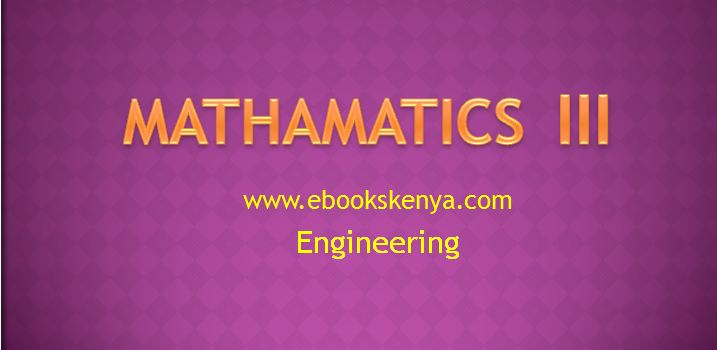# Mathematics III notes KNEC DiplomaIntroduction

This module unit is designed with knowledge, skills, techniques and attitudes necessary to enhance the trainee’s understanding of other analytical areas of study in this course. The module unit will also be very useful to trainees who aspire to further their training in this course.

This module is a build up of Mathematics I and II of this course. Trainees undertaking this module unit require to have successfully completed Mathematics I and II of this course or its equivalent.

Timed tests, assignment, end of Module examinations are the recommended mode of evaluation for this Module unit, and any other suitable method.

### Vector Field Theory

• Definition of dot and cross products of vectors
• Solution of problems involving dot and cross products of vectors
• Definition of operators
• Definition of vector field
• Definition of curl F
• Solutions of problems involving curl F
• Solutions of problems involving F

### Matrices

• Matrix operation
• Determinant of 3×3 matrix
• Inverse of 3×3 matrix
• Solution of linear simultaneous equations in 3 unknowns
• Application of matrices

### Numerical Methods

• Definition of interpolation and extrapolation
• Application of interpolation and
• Application of interactive methods to solve equations
• Application of interactive methods to areas and volumes

### Double And Triple Integrals

• Definition of double and triple integrals
• Use of multiple integrals to find areas and volume
• Consideration of double integrals in polar and cylindrical coordinates
• Use of triple integrals in solving problems

### Differential Equations

• Types of first order differential equations
• Formation of first order differential equations
• Solutions of first order differential equations
• Application of first order 8differential equations Formation of the second order differential equations for various systems
• Solution of second order differential equations ‘
• Application of second order differential equations

### Laplace Transforms

• Definition of Laplace transforms Deriving Laplace transforms from first principles
• State properties of Laplace transform
• Determination of inverse LT of simple transforms and partial fractions
• Solution of differential equations by LT
• Solution of simultaneous differential equations by given initial conditions

### Fourier series

• Determination of the fourier series as a periodic function of period 2 and extended to
• Determination of fourier series of non-perodic functions over a given range
• Determination of fourier series for even and odd functions and the half-range series for a given function

### Loci

• Definition of a point
• Locus of a point in relation to a circle
• Loci of points for given mechanism
(Visited 2,529 times, 1 visits today)

## 3 thoughts on “Mathematics III notes KNEC Diploma”

1.martin gikungi says:

it a good book please send me

2.Elvines Otieno says:

It is a good revision material, how can I get it please?

1.Bethuel naibei says:

Nice good